# ICSE Class 10 Maths Important Questions And Solutions - Free PDF Download

Mathematics is a subject that deals with the logic of shape, quantity and arrangement. Math is all around us in everything we do. Important questions of Class 10 maths is the shortest possible preparation tester available. Practising important questions for ICSE Class 10 Maths is highly beneficial because it gets the students accustomed pattern of the exam, which will eventually make the students confident. Solving these important questions will help students a lot in their school as well as board exams.

By following ICSE Class 10 Maths important questions, students can evaluate their performance and preparations so far. All the essential concepts and topics of Class 10 Maths are covered while framing the important questions to help the students to improve their conceptual learning and problem-solving skills. These important questions of Class 10 Maths are designed by subject experts referring to the ICSE Class 10 Maths Syllabus, and solving them will help the students to improve their time and approach.

## Download ICSE Class 10 Maths Important Questions & Solutions In PDF

To help students while preparing for the ICSE Class 10 Board exam, we have provided below the ICSE Class 10 Important Questions of Maths, which covers all the important numerical problems from each chapter.

Question 1:

Ben deposits a certain sum of money each month in a recurring deposit account of a bank. If the rate of interest is 8% per annum and Ben gets Rs. 8,088 from the bank after 3 years, find the value of his monthly instalment.

Solution:

Let P be the monthly instalment.

Given,

Rate of interest = r = 8%

Time = n = 36 (i.e. 3 years)

Maturity value at the end of 3 years = Rs. 8088

SI = P × [n(n + 1)/ (2 × 12)] × (r/100)

= P × [36(36 + 1)/ 24] × (8/100)

= P × (3 × 37/ 2) × (2/25)

= P × (3 × 37)/25

= 4.44P

Total amount at the time of maturity = (P × n) + SI

8088 = 36P + 4.44P

8088 = 40.44P

P = 8088/ 40.44

P = 200

Therefore, the monthly instalment is Rs. 200.

Question 2:

A bag contains 5 white balls, 6 red balls and 9 green balls. A ball is drawn at random from the bag. Find the probability that the ball drawn is:

(i) a green ball

(ii) a white or a red ball

(iii) neither a green ball nor a white ball

Solution:

Given,

A bag contains 5 white balls, 6 red balls and 9 green balls.

Total number of outcomes = n(S) = 5 + 6 + 9 = 20

(i) Let A be the event of getting a green ball.

Number of outcomes favourable to A = n(A) = 9

P(A) = n(A)/ n(S)

= 9/20

(ii) Let B be the event of getting a white or a red ball.

Number of outcomes favourable to B = n(B) = 11

i.e. 5(white) + 6(red) = 11 balls

P(B) = n(B)/ n(S)

= 11/20

(iii) Let C be the event of getting neither a green ball nor a white ball.

Number of outcomes favourable to C = n(C) = 6

i.e. Total number of balls – (green + white balls) = 20 – (9 + 5) = 6

P(C) = n(C)/ n(S)

= 6/20

= 3/10

Question 3:

Find the minimum length in cm and correct it to the nearest whole number of a thin metal sheet required to make a hollow and closed cylindrical box of diameter 20 cm and height 35 cm. The width of the metal sheet is 1 m. Also, find the cost of the sheet at the rate of Rs. 56 per m. Find the area of the metal sheet required if 10% of it is wasted in cutting, overlapping etc.

Solution:

Given,

Diameter of hollow and closed cylinder = 20 cm

Radius of the cylinder = r = 20/2 = 10 cm

Height of cylinder = h = 35 cm

Total surface area of the cylinder = 2πr(r + h)

= 2 × (22/7) × 10 × (10 + 35)

= (44/7) × 10 × 45

= 2828.57 cm2

= 0.282 m2

Width of the sheet = 1 m

Cost of sheet per m = Rs. 56

Total cost of the sheet = 0.282 × Rs. 56 = Rs. 15.8

Length of the sheet = Area of sheet/ Width of the sheet

= 0.282/ 1 = 0.282 m = 28.2 cm

Area of the metal sheet required if 10% of it is wasted in cutting, overlapping etc.,

= 2828.57 + 10% of 2828.57

= 2828.37 + 282.8

= 3111.57 cm2

Question 4

Geetha repays her total loan of 1,18,000 by paying instalments every month. If the instalment for the first month is 1,000 and it increases by 100 every month, what amount will she pay as the 30th instalment of the loan? What amount of the loan has she to still pay after the 30th instalment?

Solution:

Given,

Total loan amount = Rs. 1,18,000

First instalment = Rs. 1000

Second instalment = Rs. 1000 + Rs. 100 = Rs. 1100

Third instalment = Rs. 1100 + Rs. 100 = Rs. 1200

This is an AP with a = 1000 and d = 100

nth term of an Ap:

an = a + (n – 1)d

a30 = 1000 + (30 – 1) × 100

= 1000 + 29 × 100

= 1000 + 2900

= 3900

Therefore, the amount paid in the 30th instalment is Rs. 3900.

Sum of first n term of an AP:

Sn = n/2 [a + an]

S30 = (30/2) × (1000 + 3900)

= 15 × 4900

= 73500

Therefore, the amount paid in 30 instalments is Rs. 73,500

Loan amount to be paid after 30th instalment = Total loan amount – Amount paid in 30 instalments

= Rs. 1,18,000 – Rs. 73,500

= Rs. 44,500

Question 5

In the given figure, AD is a diameter. O is the centre of the circle. AD is parallel to BC, and ∠CBD = 32°.

Find:

(i) ∠OBD

(ii) ∠AOB

(iii) ∠BED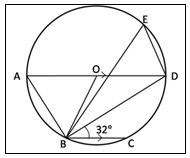Solution:

Given,

AD is the diameter of the circle with centre O.

AD is parallel to BC, and ∠CBD = 32°.
From the given figure,

(i) ∠ODB = ∠CBD (alternate interior angles)

OB = OD (radii of the same circle)

∠OBD = ∠ODB = 32°

We know that the angle in a semicircle is 90°.

∠ABD = 90°

And

∠ABO = ∠ABD – ∠OBD

= 90° – 32°

= 58°

∠ABO = 58°

Also, the angles opposite the equal sides are equal.

∠OAB = ∠OBA = 58° (OA = OB)

In triangle AOB,

∠OAB + ∠AOB + ∠OBA = 180

58° + ∠AOB + 58° = 180°

∠AOB = 180° – 58° – 58°

∠AOB = 64°

(iii) Angles in the same segment are equal.

Question 6

Prove that: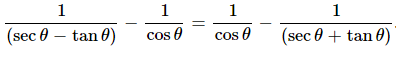Solution:

LHS = [1/ (sec θ – tan θ)] – (1/cos θ)

Using the identity sec2A – tan2A = 1,

= [(sec2θ – tan2θ) / (sec θ – tan θ)] – sec θ

= [(sec θ + tan θ) (sec θ – tan θ) / (sec θ – tan θ)] – sec θ

= sec θ + tan θ – sec θ

= tan θ….(i)

RHS = (1/cos θ) – [1/ (sec θ + tan θ)]

= sec θ – [(sec2θ – tan2θ) / (sec θ + tan θ)]

= sec θ – [(sec θ + tan θ) (sec θ – tan θ) / (sec θ + tan θ)]

= sec θ – sec θ + tan θ

= tan θ….(ii)

From (i) and (ii),

LHS = RHS

Hence proved.

Question 7

Prove by factor theorem that

(i) (x – 2) is a factor of 2x3 – x2 – 7x + 2

(ii) (2x + 1) is a factor of 4x3 + 12x2 + 7x +1

(iii) (3x – 2) is a factor of 18x3 – 3x2 + 6x – 8

Solution:

(i) Let p(x) = 2x3 – x2 – 7x + 2

For checking, (x – 2) is a factor of p(x); substitute x = 2 in p(x).

p(2) = 2(2)3 – (2)2 – 7(2) + 2

= 2(8) – 4 – 14 + 2

= 16 – 18 + 2

= 0

Therefore, (x – 2) is a factor of 2x3 – x2 – 7x + 2.

(ii) Let p(x) = 4x3 + 12x2 + 7x +1

For checking, (2x + 1) is a factor of p(x); substitute x = -1/2 in p(x).

p(-½) = 4(-½)3 + 12(-½)2 + 7(-½) + 1

= 4(-⅛) + 12(¼) – (7/2) + 1

= (-½) + 3 – (7/2) + 1

= (-8/2) + 4

= -4 + 4

= 0

Therefore, (2x + 1) is a factor of 4x3 + 12x2 + 7x +1.

(iii) Let p(x) = 18x3 – 3x2 + 6x – 8

For checking, (3x – 2) is a factor of p(x); substitute x = 2/3 in p(x).

p(⅔) = 18(⅔)3 – 3(⅔)2 + 6(⅔) – 12

= 18(8/27) – 3(4/9) + 4 – 8

= (16/3) – (4/3) – 4

= (12/3) – 4

= 4 – 4

= 0

Question 8

Draw an Ogive for the following distribution:

 Class 0 – 10 10 – 20 20 – 30 30 – 40 40 – 50 50 – 60 60 – 70 70 – 80 Frequency 6 10 18 22 20 15 6 3

Find the median and interquartile range from the obtained ogive.

Solution:

Cumulative frequency distribution table:

 Class Frequency Cumulative frequency 0 – 10 6 6 10 – 20 10 16 20 – 30 18 34 30 – 40 22 56 40 – 50 20 76 50 – 60 15 91 60 – 70 6 97 70 – 80 3 100

N/2 = 100/ 2

Ogive: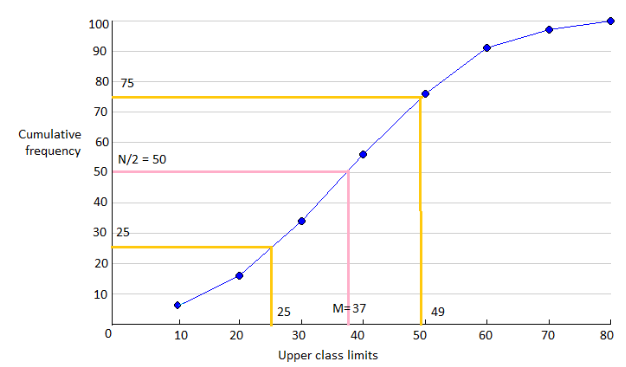Median = 37

Lower quartile = (N/4)th observation = (100/4) = 25th observation = 25

Upper quartile = (3N/4)th observation = (300/4) = 75th observation = 49

Interquartile range = Upper quartile – Lower quartile

= 49 – 25

= 24

Question 9

Solve the following inequation.

-2 2/3 ≤ x + (1/3) < 3 + (1/3), x ∈ R

Represent the solution set on the number line.

Solution:

-2 2/3 ≤ x + (1/3) < 3 + (1/3)

-(8/3) ≤ x + (1/3) < (10/3)

(-8/3) – (1/3) ≤ x < (10/3) – (1/3)

-9/3 ≤ x < 9/3

-3 ≤ x < 3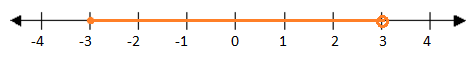The open circle at 3 indicates that 3 is not included in the solution set.

Question 10

In the given figure. Line AB meets the y-axis at point A. The line through C(2, 10) and D intersects line AB at a right angle at point P. Find:

(i) equation of line AB

(ii) equation of line CD

(iii) coordinates of points E and D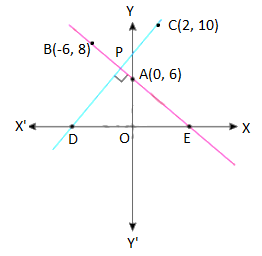Solution:

From the given figure,

A = (0, 6)

B = (-6, 8)

C = (2, 10)

(i) Slope of line AB = (8 – 6)/ (-6 – 0) = -2/6 = -1/3

i.e. m = -1/3

y-intercept of the line AB = 6

Equation of the line in slope intercept form is y = mx + c

y = (-1/3)x + 6

y = (-x + 18)/3

3y = -x + 18

x + 3y – 18 = 0

(ii) Given,

AB and CD interest at right angles.

Slope of AB × Slope of CD = -1

(-1/3) × Slope of CD = -1

Slope of CD = -1 × (-3/1) = 3

Equation of the line with slope 3 and passing through the point C(2, 10) is:

y – 10 = 3(x – 2)

y – 10 = 3x – 6

3x – 6 – y + 10 = 0

3x – y + 4 = 0

Therefore, the equation of line CD is 3x – y + 4 = 0.

(iii) Point E lies on the line AB and on the x-axis.

The y-coordinate of E is 0.

Let the coordinates of E be (x, 0).

Substituting E(x, 0) in the equation of line AB.

x + 3(0) – 18 = 0

x = 18

Thus, E = (18, 0)

Similarly,

y-coordinate of D is 0.

Substituting D in the equation of line CD.

3x – 0 + 4 = 0

3x = -4

x = -4/3

Therefore, the coordinates of D are (-4/3, 0).

Question 11

Find a GP for which the sum of the first two terms is -4, and the fifth term is 4 times the third term.

Solution:

Let a be the first term and r be the common ratio of a GP.

nth term of a GP = an = arn – 1

Given,

a1 + a2 = -4

a + ar = -4

a(1 + r) = -4….(i)

And

a5 = 4a3

ar(5 – 1) = 4 × ar(3 – 1)

r4 = 4r2

r2 = 4

r = ± 2

When r = 2,

a(1 + 2) = -4  [From (i)]

3a = -4

a = -4/3

ar = (-4/3) × 2 = -8/3

ar2 = (-4/3) × (2)2 = -16/3

When r = -2,

a(1 – 2) = -4

-a = -4

a = 4

ar = 4(-2) = -8

ar2 = 4(-2)2 = 4 × 4 = 16

Therefore, the required GP is -4/3, -8/3, -16/3,…. Or 4, -8, 16,…

Question 12

A man invests a certain sum on buying 15% Rs. 100 shares at a 20% premium. Find:

(i) His income from one share

(ii) The number of shares bought to have an income from the dividend as Rs. 6480

(iii) The sum invested.

Solution:

(i) Dividend on one share = 15% of Rs. 100

= (15/100) × Rs. 100

= Rs. 15

Therefore, the income from one share is Rs. 15.

(ii) The number of shares bought to have an income from the dividend as Rs. 6480

= Annual income/ Dividend on one share

= 6480/ 15

= Rs. 432

(iii) Given that the man bought shares of Rs 100 at a 20% premium, the market value of one share

= (120/100) × Rs. 100

= Rs. 120

Total investment = Number of shares x Market value of one share

= 432 × Rs. 120

= Rs. 51,840

Question 13

Attempt this question on a graph paper.

(i) Plot A (3, 2) and B (5, 4) on a graph paper. Take 2 cm = 1 unit on both axes.

(ii) Reflect A and B in the x-axis to A’ and B’, respectively. Plot these points also on the same graph paper.

(iii) Write down:

(a) the geometrical name of the figure ABB’A’

(b) the measure of angle ABB’

(c) the image of A” of A when A is reflected in the origin

(d) the single transformation that maps A’ to A”.

Solution:

Given,

A (3, 2) and B (5, 4)

A’ and B’ are the reflections of A and B, respectively, in the x-axis.

A” is the image of A when A is reflected in the origin.

(i)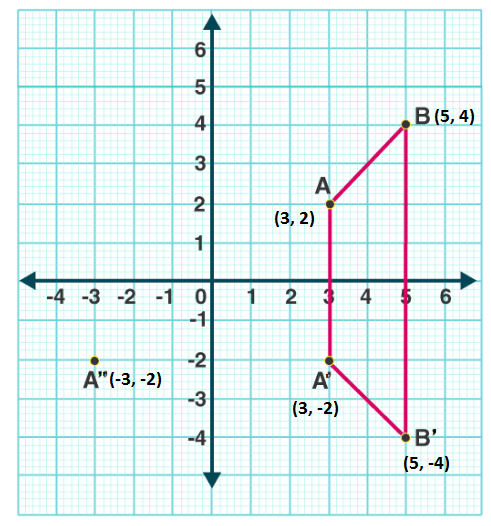(ii) Coordinates of A'(3, -2) and the coordinates of B'(5, -4).

(iii)

(a) ABB’A’ is an isosceles trapezium.

(b) The measure of angle ABB’ is 45°.

(c) Coordinates of A” = (-3, -2)

(d) The single transformation that maps A’ to A” is the reflection in the y-axis.

Question 14

A school has 630 students. The ratio of the number of boys to the number of girls is 3: 2. This ratio changes to 7: 5 after the admission of 90 new students. Find the number of newly admitted boys.

Solution:

Given,

Total number of students = 630

The ratio of the number of boys to the number of girls is 3: 2.

Let 3x be the number of boys and 2x be the number of girls.

⇒ 3x + 2x = 630

5x = 630

x = 630/5

x = 126

Number of boys = 3x = 3 × 126 = 378

Number of girls = 2x = 2 × 126 = 252

After the admission of 90 new students, the ratio of boys to girls is 7: 5. (given)

Total number of students = 630 + 90 = 720

Let 7x be the number of boys, and 5x be the number of girls.

⇒ 7x + 5x = 720

12x = 720

x = 720/12

x = 60

The number of boys = 7x = 7 × 60 = 420

The number of girls = 5x = 5 × 60 = 300

Therefore, the number of newly admitted boys = 420 – 378 = 42

Question 15

Prove: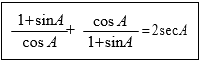Solution:

LHS = [(1 + sin A)/ cos A] + [cos A/ (1 + sin A)]

= [(1 + sin A)2 + cos2A] / [(1 + sin A) cos A]

= [1 + sin2A + 2 sin A + cos2A] / [(1 + sin A) cos A]

= (1 + 1 + 2 sin A) / [(1 + sin A) cos A]

= (2 + 2 sin A)/ [(1 + sin A) cos A]

= [2(1 + sin A)] / [(1 + sin A) cos A]

= 2/cos A

= 2 sec A

= RHS

Hence proved.

Question 16

Solve for x using the quadratic formula. Write your answer correct to the two significant figures. (x – 1)2 – 3x + 4 = 0

Solution:

Given,

(x – 1)2 – 3x + 4 = 0

x2 + 1 – 2x – 3x + 4 = 0

x2 – 5x + 5 = 0

Comparing with the standard form ax2 + bx + c = 0,

a = 1, b = -5, c = 5

x = [-b ± √(b2 – 4ac)] / 2a

= [-(-5) ± √{(-5)2 – 4(1)(5)}] / 2(1)

= [5 ± √(25 – 20)]/ 2

= (5 ± √5)/2

= (5 ± 2.24)/2

x = (5 + 2.24)/2, x = (5 – 2.24)/2

x = 7.24/2, x = 2.76/2

x = 3.62, x = 1.38

Question 17

In the given figure, DE ‖ BC, AE = 15 cm, EC = 9 cm, NC = 6 cm and BN = 24 cm. Find the lengths of ME and DM.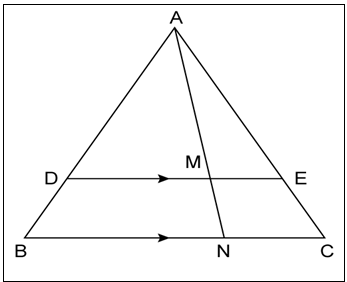Solution:

Given,

DE ‖ BC, AE = 15 cm, EC = 9 cm, NC = 6 cm and BN = 24 cm.

From the given figure,

ME || NC

In Δ AME and Δ ANC,

∠AME = ∠ANC

∠MAE = ∠NAC (common angle)

By AA similarity criterion,

∆AME ~ ∆ANC

We know that the corresponding sides of similar triangles are proportional.

ME/NC = AE/AC

ME/ 6 = 15/ 24

ME = 3.75 cm

In Δ ADM and Δ ABN,

∠ADM = ∠ABN ( DE || BC so, DM || BN)

∠DAM = ∠BAN (common angle)

By AA similarity criterion,

In Δ ADE and Δ ABC,

∠ADE = ∠ABC (since DE || BC so, ME || NC)

∠AED = ∠ACB

By AA similarity criterion,

⇒ AD/AB = AE/AC = 15/24 …. (i)

⇒ DM/BN = AD/AB = 15/24 [From (i)]

DM/ 24 = 15/ 24

DM = 15 cm

Therefore, ME = 3.75 cm and DM = 15 cm.

Question 18

One pipe can fill a cistern in 3 hours less than the other. The two pipes together can fill the cistern in 6 hours 40 minutes. Find the time that each pipe will take to fill the cistern.

Solution:

Let one pipe fill the cistern in x hours, and the other fills the cistern in (x – 3) hours.

Given,

The two pipes together can fill the cistern in 6 hours 40 minutes.

i.e. 6 2/3 hours = 20/3 hours

⇒ (1/x) + [1/ (x – 3)] = 3/20

⇒ (x – 3 + x)/ [x(x – 3)] = 3/20

⇒ (2x – 3)/ (x2 – 3x) = 3/20

⇒ 20(2x – 3) = 3(x2 – 3x)

⇒ 40x – 60 = 3x2 – 9x

⇒ 3x2 – 9x – 40x + 60 = 0

⇒ 3x2 – 49x + 60 = 0

⇒ 3x2 – 45x – 4x + 60 = 0

⇒ 3x(x – 15) – 4(x – 15) = 0

⇒ (3x – 4)(x – 15) = 0

⇒ 3x – 4 = 0, x – 15 = 0

⇒ x = 4/3, x = 15

Now,

x – 3 = (4/3) – 3 = -5/4 (not possible)

Therefore, x = 15

x – 3 = 15 – 3 = 12

Hence, one pipe can fill the cistern in 15 hours and the other in 12 hours.

Question 19

A circus tent is in the shape of a cylinder surmounted by a conical top of the same diameter. If their common diameter is 56 m, the height of the cylindrical part is 6 m, and the total height of the tent above the ground is 27 m. Find the area of the canvas used in making the tent.

Solution:

Given,

Total height of the tent = 27 m

Diameter of the circular bases = 56 m

Radius of cylinder = Radius of conical top = r = 56/2 = 28 m

Height of the cylinder = h = 6 m

Height of the conical part = H = 27 – h = 27 – 6 = 21 m

Slant height of the conical part = √(r2 + h2)

l = √[(28)2 + (21)2]

= √(784 + 441)

= √1225

= 35 m

Area of the canvas used = CSA of cylinder + CSA of cone

= 2πrh + πrl

= πr(2h + l)

= (22/7) × 28 × (2 × 6 + 35)

= 22 × 4 × (12 + 35)

= 88 × 47

= 4136 m2

Therefore, the area of the canvas used in making the tent is 4136 m2.

Question 20

Ankit deposits Rs 2,250 per month in a recurring deposit account for a period of 3 years. At the time of maturity, he will get Rs 90,990.

(i) Find the rate of simple interest per annum

(ii) Find the total interest earned by Ankit.

Solution:

Given,

Monthly instalment = P = Rs. 2250

Time = n = 36 months (i.e. 3 years)

Amount at the time of maturity = Rs. 90,990

Let r be the rate of interest.

Total amount deposited = Rs. 2250 × 36 = Rs. 81,000

Interest = Amount at the time of maturity – Total amount deposited

= Rs. 90,990 – Rs. 81,000

= Rs. 9990

SI = P × [n(n + 1)/ (2 × 12)] × (r/100)

9990 = 2250 × (36 × 37/ 24) × (r/100)

9990 = 2250 × (3 × 37) × (r/200)

r = (9990 × 200)/ (2250 × 3 × 37)

r = 8

Therefore, the rate of interest is 8%, and the total interest earned by Ankit is Rs. 9990.

Question 21

Ms. Kumar has an account in ICICI Bank. Entries of his passbook has given below:

 Date, 2008 Particulars Withdrawals (in Rs.) Deposits (in Rs.) Balance (in Rs.) Jan 3 B/F _ _ 2642 Jan 16 To self 640 _ 2002 March 4 By cash _ 850 2852 April 10 To self 1130 _ 1722 April 25 By cheque _ 650 2372 June 15 By cash 577 _ 1795

Calculate the total amount received by Kumar if he closes his account on August 1, 2008, and the rate of interest is 5% per annum.

Solution:

Qualifying amount on a monthly basis:

Jan = 2002

Feb = 2002

March = 2852

April = 1722

May = 2372

June = 1795

July = 1795

Total = Rs. 14540

Let the principal amount for one month = Rs. 14540

Rate of interest = 5%

Interest = (14540 × 1 × 5)/ (12 × 100)

= Rs. 60.58

The total amount received by Kumar if he closes his account on August 1, 2008

= Balance at the end of July + Interest earned from Jan to July

= Rs. 1795 + Rs. 60.58

= Rs. 1855.58

### Advantages of solving ICSE Class 10 Maths Important Questions

• Solving these important questions will make them feel confident.
• It is considered the most valuable resource for revising the entire syllabus in a quick time.
• By going through the ICSE important questions, students can self-analyze their performance.
• It also helps to clear their concepts and doubts.